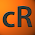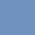## Pic## Wednesday, October 23, 2013

### Squaring and Cubing as the Bedrock of Exponentiation

Can anyone show me an example of exponentiation being introduced to our shortest students as anything by repeated-multiplication?

We know that exponentiation is hard for kids. Part of this is because, well, exponentiation is hard. But take a close look at exponents mistakes, and multiplication often ends up looking like the culprit.

Exponentiation isn't necessarily benefiting from its definition as repeated multiplication.

Professor Danielson suggested that we remedy the situation by introducing exponents in terms of doublings and triplings and the like:
"What if we think of the powers of 2 not as repeated multiplication, but as the number of doublings?"
Though he recently offered an update...

So what else can we do?

My idea is that we build exponentiation on top of rock-solid concepts of squaring and cubing. We start as young as third or fourth grade, where we offer kids squares of various side-lengths and ask them how many unit squares it's composed of. Then we offer them cubes a side-length and ask them to find the number of unit cubes. Eventually, we call this "squaring" and "cubing" without ever using the power notation. Then, in later work, we'll introduce exponentiation as an extension of the concepts of squaring and cubing.

The value of these sorts of problems on their own is immense. First, they connect well with the array work that the kids are hopefully doing. They also drive at the connection between area and volume, and constitute good multiplication practice. We're also helping set them up for square roots and cube roots.

But I see this as crucial in setting up exponentiation. First, because we'll make sure to connect these sorts of problems with the language of squaring and cubing. (None of my 5th Graders knew why squaring was called squaring, FYI.) The second reason why this is crucial is because, again, we're going to introduce exponentiation as an extension of squaring and cubing. This means that students will have a rock solid example to pivot off of as they learn fourth, fifth and other powers. Third, it avoids using first powers in the early development of the exponentiation concept, which might very well be the seed of many future exponent/multiplication mix ups.

I want to be briefish, so I'll say that I tried this out with a group of 5th Graders this week. They had all seen exponentiation before, but were incredibly confused by it. Many were writing "12" for 3^4. None of them knew how the language of squaring and cubing related to powers.

I started class by asking a variety of squaring and cubing problems. These were of medium difficulty for the kids, but eventually we noticed that a cube is made up of X sheets that have X-squared cubes, leading the kids to suggest a generalization: "a number cubed is always the number squared times that number."

It felt natural to go around and remind the kids that 3^4 was like 3-squared or 3-cubed, but to the fourth power. One thing that was frustrating was that a bunch of kids didn't see why 3^2 should be the same thing as "three squared." But I think that's a frustration that I could avoid if I had the chance to lay the groundwork of that kid's concept of exponents.

So, that's my idea. Thoughts?

Update: After a second round trying to help out some 5th Graders, I realized that it's not enough to just deal with 2D squares and 3D cubes. There needs to be a middle stage where we're saying that "squaring" three means building a square out of a length of three cubes. Otherwise the language of "Three cubed is three squares" doesn't really work.

Also, kids today asked whether there was such a thing as "three triangled," which is a great example of a question as evidence of learning.

1.Please tell you've seen this.

1.2.2.One thing I'm wondering about is the directionality of squaring vs. exponentiating, and the question of what's constant. I asked Christopher about this too.

On the one hand, raising to a power is an operation. 2^3 takes 2 and 3 and maps them to 8, just like 2 * 3 takes 2 and 3 and maps them to 6.

On the other hand, operations are usually friendly, associative, commutative things, so that 2 * 3 maps to the same thing as 3 * 2, and thus the operation doesn't have a "handedness".

But with raising to a power, 2^3 is very different that 3^2.

When kids meet squaring, it's usually in the context of "What's 3 squared? How about 4 squared? What about 5 squared? 10 squared?" The function they're playing with is x^2, and they're thinking about take any number and apply the squaring function.

When they meet exponentiation, at least later on in Algebra, they're thinking about "What's 2^3? How about 2^4? 2^5? 2^10?" and it's all about doublings. That squaring and doubling are two sides of the same operation may be lost on them at first... but I'm not sure I have a problem with that. I want them to resolve that ambiguity eventually, but I'd be willing to bet that studying squaring and studying exponential growth were wildly different fields in terms of historical development [refutable hypothesis 1].

My other refutable hypothesis is that kids who meet exponentiation and play around with both lots of 2^? and ?^2 at near the same time, will overgeneralize commutativity -- not necessarily a bad thing, but one that teachers will need to be deliberately on the lookout for. Not only is 2^? not twice ?, it's also not the same as ?^2.

Good questions! Please report back on your 5th graders' continued exploring!

3.There's a lot of deep and useful stuff here. But I think that dispensing with the notion of exponentiation as repeated multiplication is throwing the baby out with the bathwater. Repeated multiplication

(1) Is easy to explain and understand.
(2) Gives a clear meaning to the notation.
(3) Leads directly into the properties of exponentiation (e.g., a^b*a^c = a^(b+c)), from which you can later generalize.

The belief that 2^3 means 2*3 strikes me as pretty quick to fix. I'm not sure it's worth getting off the highway, and taking backroads towards teaching exponentiation, just because we see brakelights on a few cars.